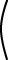``` 10 0 20 20 40 10 0 20 10 ```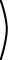``` -2 2 1 1 2 -2 ```= 10``` 1 0 2 2 4 1 0 2 1 `````` -2 2 1 1 2 -2 ```= 10``` 2 -2 2 6 4 0 ```= 20``` 1 -1 1 3 2 0 ```# Associative

In the following, multiply the first two matrices together. Click on the = to check your result. Then multiply the result with the third matrix.

Problem Partial Result Result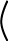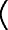``` 1 -1 2 3 ```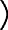``` -2 1 0 2 ```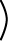``` -1 0 1 1 ```? ? ? ?``` -1 0 1 1 ```? ? ? ?Now do the problem again, but this time start with the last two matrices.

Problem Partial Result Result``` 1 -1 2 3 `````` -2 1 0 2 `````` -1 0 1 1 `````` 1 -1 2 3 ```? ? ? ?? ? ? ?The final answer is the same for both ways of doing the problem. This demonstates that matrix multiplication is associative:

(AB)C  =  A(BC)

Of course, the inner dimensions of A and B must be the same, and the inner dimensions of B and C must be the same. Usually a product of three matrices is written ABC.

### QUESTION 11:

Say that  5×5  B ?×?3×4  =  ?×?

What are the dimensions of B and D ?